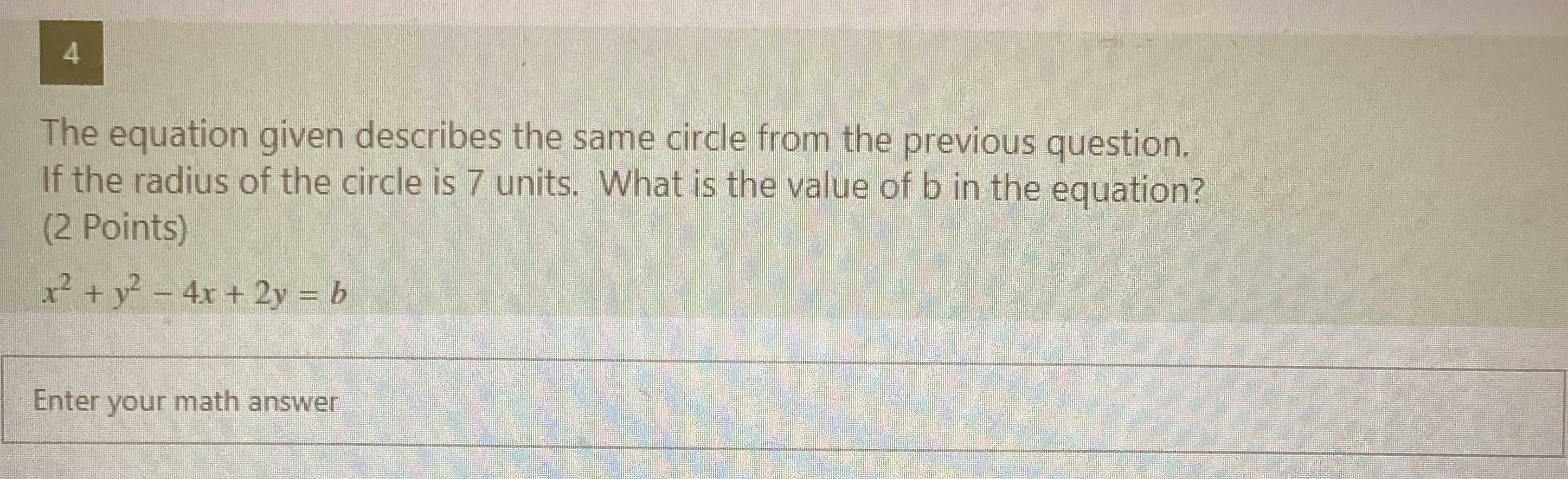### ¿Todavía tienes preguntas de matemáticas?

Pregunte a nuestros tutores expertos
Algebra
Pregunta$$4$$ The equation given describes the same circle from the previous question. If the radius of the circle is $$7$$ units. What is the value of b in the equation? (2 Points) $$x ^ { 2 } + y ^ { 2 } - 4 x + 2 y = b$$

$$b= 54$$